Open in App
Not now

# Select row with maximum and minimum value in Pandas dataframe

• Last Updated : 07 Sep, 2022

Let’s see how can we select rows with maximum and minimum values in Pandas Dataframe with help of different examples using Python.

## Python3

 `# importing pandas and numpy` `import` `pandas as pd` `import` `numpy as np`   `# data of 2018 drivers world championship` `dict1 ``=` `{``'Driver'``: [``'Hamilton'``, ``'Vettel'``, ``'Raikkonen'``,` `                    ``'Verstappen'``, ``'Bottas'``, ``'Ricciardo'``,` `                    ``'Hulkenberg'``, ``'Perez'``, ``'Magnussen'``,` `                    ``'Sainz'``, ``'Alonso'``, ``'Ocon'``, ``'Leclerc'``,` `                    ``'Grosjean'``, ``'Gasly'``, ``'Vandoorne'``,` `                    ``'Ericsson'``, ``'Stroll'``, ``'Hartley'``, ``'Sirotkin'``],`   `         ``'Points'``: [``408``, ``320``, ``251``, ``249``, ``247``, ``170``, ``69``, ``62``, ``56``,` `                    ``53``, ``50``, ``49``, ``39``, ``37``, ``29``, ``12``, ``9``, ``6``, ``4``, ``1``],`   `         ``'Age'``: [``33``, ``31``, ``39``, ``21``, ``29``, ``29``, ``31``, ``28``, ``26``, ``24``, ``37``,` `                 ``22``, ``21``, ``32``, ``22``, ``26``, ``28``, ``20``, ``29``, ``23``]}`   `# creating dataframe using DataFrame constructor` `df ``=` `pd.DataFrame(dict1)` `print``(df.head(``10``))`

Output: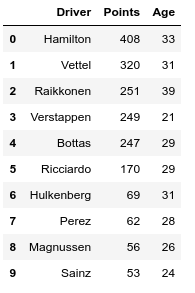## Select row with maximum value in Pandas Dataframe

Example 1: Shows max on Driver, Points, and Age columns.

## Python3

 `# creating dataframe using DataFrame constructor` `df ``=` `pd.DataFrame(dict1)`   `# the result shows max on` `# Driver, Points, Age columns.` `print``(df.``max``())`

Output: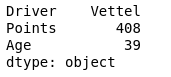Example 2: Who scored max points

## Python3

 `# creating dataframe using DataFrame constructor` `df ``=` `pd.DataFrame(dict1)`   `# Who scored more points ?` `print``(df[df.Points ``=``=` `df.Points.``max``()])`

Output: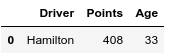Example 3: What is the maximum age

## Python3

 `# creating dataframe using DataFrame constructor` `df ``=` `pd.DataFrame(dict1)`   `# what is the maximum age ?` `print``(df.Age.``max``())`

Output:

`39`

Example 4: Which row has maximum age in the Dataframe | who is the oldest driver?

## Python3

 `# creating dataframe using DataFrame constructor` `df ``=` `pd.DataFrame(dict1)`   `# Which row has maximum age |` `# who is the oldest driver ?` `print``(df[df.Age ``=``=` `df.Age.``max``()])`

Output: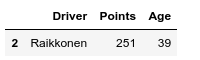## Select row with maximum value in Pandas Dataframe

Example 1: Shows min on Driver, Points, Age columns.

## Python3

 `# creating dataframe using DataFrame constructor` `df ``=` `pd.DataFrame(dict1)`   `# the result shows min on` `# Driver, Points, Age columns.` `print``(df.``min``())`

Output: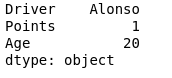Example 2: Who scored fewer points

## Python3

 `# creating dataframe using DataFrame constructor` `df ``=` `pd.DataFrame(dict1)`   `# Who scored less points ?` `print``(df[df.Points ``=``=` `df.Points.``min``()])`

Output: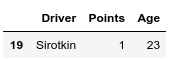Example 3: Which row has minimum age in the Dataframe who is the youngest driver

## Python3

 `# creating dataframe using DataFrame constructor` `df ``=` `pd.DataFrame(dict1)`   `# Which row has maximum age |` `# who is the youngest driver ?` `print``(df[df.Age ``=``=` `df.Age.``min``()])`

Output: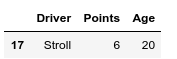My Personal Notes arrow_drop_up
Related Articles